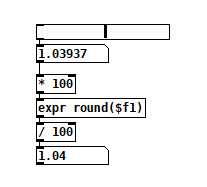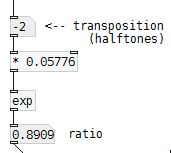• ### BPM/Pitch calculator

BPMcalculator.pd

Messing about with this simple patch to calculate BPM change when something is slowed down two semitones and I wondered - how would I find the most whole numbered/least amount of decimal places change/ratio?

I think this is probably a basic maths question, but I have no clue how to approach it. Thanks!

• Posts 14 | Views 4023
• Thanks - but the maths help I need is how to find ratios between the bpm changes.

• @lead A semitone is 1/12 of an octave, so if you raise the pitch by a semitone, you're raising the tempo by 2 to the power of 1/12 and would multiply the tempo by that.
So if you lower the pitch by 2 semitones you would divide the tempo by 2 to the power of 2/12
The tempo ratio (old to new) would be 1 : (1 / 2 to the power of 0.166666667)
The tempo ratio (new to old) would be (1 / 2^0.166666667) : 1
As sure as I can be...

You can do the math in your patch using [pow]
David.

• Have you had a look at the patch I posted? I think that’s what I did!

I suppose I’m looking for the most ‘harmonic’ ratios. Would be useful going forward too I suppose when working with anharmonic spectral stuff.

• @lead I'm not sure what you're looking for, can you give us some numeric examples? If you simply want to reduce the precision of a float to some number of decimal places, you could do it this way:• @lead The ratios will be constant for semi-tones regardless of BPM (the "ratio" thing).
Sorry, I didn't see your patch (I always miss them when they are at the top of the post).
Try this........ bpm_ratio.pd
The power needs to stay positive and then is multiplied for "up" and divided for "down".
The ratios you get will keep you in tune while trying to get integers will not (except for octaves of course)..
If you want to detune then remove [int].

Screenshot below is a simpler patch but very slightly less accurate... taken from G09.pitchshift in the Pd examples.....
David.• @jameslo Two years later but hey - ratio was probably the wrong word to use, sorry. I updated the patch so it starts with whole numbers, hopefully making it easier to understand:

bpmcalculator2.pd

So good ones would be:
69 BPM gives 61.472
59 BPM gives 52.563

77 BPM gives 68.5992

It's for a studio project where I have to pitch and slow everything down 2 semitones. The starting point isn't so important, but the nearer to a whole number it is the better precision there is with keeping everything on the grid.
So, starting with the smallest number of decimal places and ending with the smallest number of decimal places is preferable, does that make sense?

• @lead Sorry, still not quite understanding what you're after. Is the 77 BPM example bad because it has 4 decimal places instead of 3 like your good examples? Or are you asking "which BPMs when pitched down 2 semitones results in a BPM that has as few decimal places as possible?" It would be cool if you were asking https://math.stackexchange.com/questions/2438510/can-i-find-the-closest-rational-to-any-given-real-if-i-assume-that-the-denominaSo, starting with the smallest number of decimal places and ending with the smallest number of decimal places is preferable, does that make sense?

Formally, it doesn't make sense.

The ratio for 2 semitones down is `2 ** (-2 / 12)`. 2 is a prime number. Raising any (positive) prime number to a fractional power results in an irrational number, with infinitely many decimal places (without ending up in a repeating sequence).

A rational number times an irrational number must be irrational. So your initial bpm value * the ratio is irrational and has infinitely many decimal places. To say "this one has 3 places" glosses over the real situation.

What you're really doing is rounding this irrational number to an arbitrary number of places. The denominator of the rounded number will be `10 ** num_places` -- thus both the numerator and denominator are integers and the result is rational.

The difference (or quotient, depending how you want to measure it) between `bpm * ratio` and the rounded version is an error value.

And the math problem, then, is to minimize the error.

You can see it more clearly if you use a language with double precision floats, e.g., SuperCollider:

``````f = { |bpm, semitones = -2, places = 3|
var r = 2 ** (semitones / 12);  // '.midiratio'
var bpm2 = bpm * r;
var rounded = bpm2.round(10 ** places.neg);
// for easier comparison I'll "absolute-value" the error values
var errorDiff = bpm2 absdif: rounded;
var errorRatio = bpm2 / rounded;
if(errorRatio < 1) { errorRatio = 1 / errorRatio };
[bpm2, errorDiff, errorRatio]
};

f.value(69);  // [ 61.472011551683, 1.1551683407163e-05, 1.0000001879178 ]
f.value(59);  // [ 52.56302437028, 2.4370280016228e-05, 1.0000004636394 ]
f.value(77);  // [ 68.599201296806, 0.00020129680612513, 1.0000029343985 ]
``````

... where, indeed, the error for 77 * r is about an order of magnitude worse.

@jameslo -- "It would be cool if you were asking https://math.stackexchange.com/questions/2438510/can-i-find-the-closest-rational-to-any-given-real-if-i-assume-that-the-denomina "

I think this is exactly what the problem reduces to -- what is the closest rational to `x` where the denominator = 1000. (However, the numerical methods in the thread will likely evaluate better with double precision floats. Pd prints 6 digits so it may not be clear what you're seeing.)

Actually something else... if x is the original bpm and y is the adjusted, find x and y where the error is minimized. So far the assumption is that x is an integer, but maybe the error could be even lower if they're both fractions.

hjh

• @jameslo Thanks for replying after such a long time, it seems I wasn't asking the right question in the first place.

@ddw_music Thank you for taking the time to write such a detailed response, I think I finally understand.

So what I actually mean is I want to minimise the error in a BPM change of two semitones, and find the start and end values where it's lowest, even if they're both fractions. I think?

So what I actually mean is I want to minimise the error in a BPM change of two semitones, and find the start and end values where it's lowest, even if they're both fractions. I think?

Yes -- you'd have an error value for the starting bpm, and another error value for `bpm * ratio`, and you would want to minimize the sum.

The tricky thing mathematically is that the error function is piecewise -- every time you jump to the next round in point, it's a different segment. So calculus-based methods for continuous functions couldn't be used directly.

Playing around with it in SuperCollider, though (not Pd, for two reasons: 1/ SC has double-precision floats, 2/ Pd is clumsy at array math):

``````~error = { |a, b, factor = 0.001|
(a absdif: a.round(factor))
+
(b absdif: b.round(factor))
};
r = -2.midiratio;

f = 69;

// let's test 0.005 bpm on either side .. 1001 total
a = (f - 0.005, f - 0.00499 .. f + 0.005);

b = a * r;  // slower bpms

c = ~error.(a, b);  // SC auto-expands math ops to arrays!

c.plot;

c.minIndex;  // 500
a[c.minIndex]  // 68.999999999994 --> 69
``````

So your "good" one is as good as that range is going to get.

Now, if you do the same thing for f = 77, the minIndex is 700 -- not 500 in the middle! -- and a[c.minIndex] = 77.001999999992 or basically 77.002.

Then:

((77.002 * r) absdif: round(77.002 * r, 0.001)) = 1.6905725900074e-05

((77.000 * r) absdif: round(77.000 * r, 0.001)) = 0.00020129683781533

So that shift of 0.002 bpm makes a huge difference.

This test also shows there's no benefit in checking "original" bpm that are not rounded to 0.001. So change it to `a = (f - 0.005, f - 0.004 .. f + 0.005);`. EDIT: In that case, the "a" term in the ~error function will be 0 (if factor = 0.001) so you could simplify to:

``````~error = { |a, factor = 0.001|
a absdif: a.round(factor)
};

// and...
c = ~error.(b);
``````

... which then does come back around to the problem that jameslo linked.

It's a brute-force technique but should work for the purpose.

hjh

• @ddw_music Amazing, thanks so much - I'll try building in SuperCollider

• Just curious: say your new BPM is off by 0.001 and around 120. I think 0.001 is a lot bigger than anything you've been discussing, but I could be wrong. Anyway, doesn't that mean that over 10 minutes, your beat will be off by 1/100ths of a quarter note, and the pitch will have been off by less than 2/100ths of a cent? (I'm glad they didn't demand that kind of precision when I was in music school!)

Posts 14 | Views 4023
Internal error.

Oops! Looks like something went wrong!